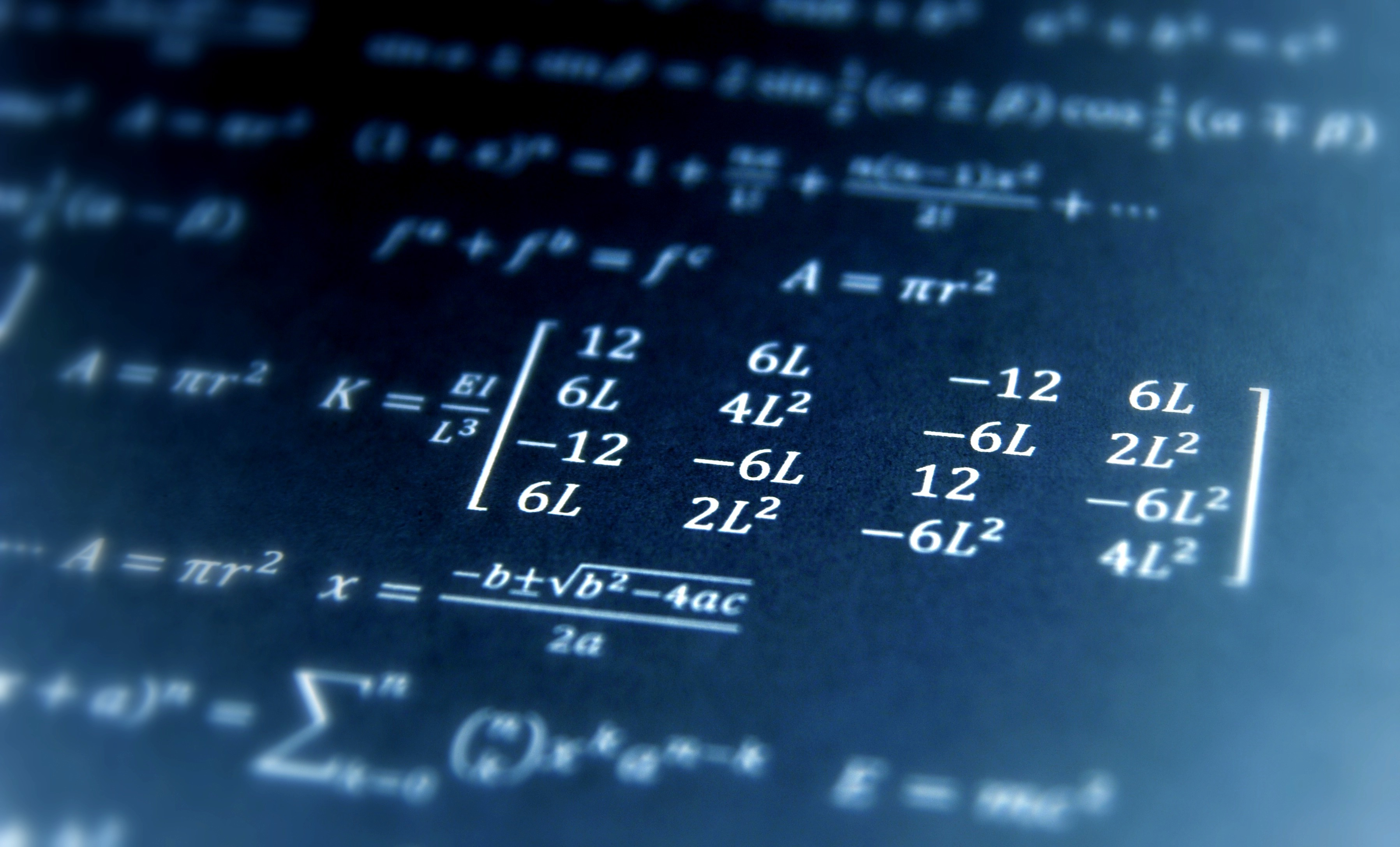# Sum of all sub matrices in 2D matrixProblem:

You need to write an algorithm to create a new 2D matrix (say matrix B of size m x n) from a given 2D matrix (say matrix A of size m x n) as an input such that the sub matrix can be represented as:

B(i,j) = ∑A(p,q) such that p=[0,i] and q=[0,j]

For Example:

If input matrix A is:

1     2     3

4     5     6

7     8     9

so the output matrix should be:

1     3     6

5   12   21

12  27  45

Solution:

Although this problem can be solved by brute force with O((mn)2) by iterating over each sub matrix in matrix A for each (i,j) in matrix B.

Our aim is to find a solution that is the most optimum solution.

Since for each (i,j) in matrix B, B(i,j) is the sum of all the elements in the sub matrix in matrix A(i,j). So we can represent matrix B such that

B(i,j) = B(i-1,j) + B(i,j-1) – B(i-1,j-1) + A(i,j)

Below is the code for the same.

```int[][] calculateMatrix(int[][] matrixA, int rows, int cols) {
int[][] matrixB = new int[rows][cols];
for (int i=0; i<rows; i++) {
for (int j=0; j<cols; j++) {
int leftEl = 0;
int topEl = 0;
int diagonalEl = 0;
if (j-1 >= 0) {
leftEl = B[i][j-1];
}
if (i-1 >= 0) {
topEl = B[i-1][j];
}
if (i-1 >= 0 && j-1 >= 0) {
diagonalEl = B[i-1][j-1];
}

matrixB[i][j] = leftEl + topEl - diagonalEl + matrixA[i][j];
}
}
return matrixB;
}```

The complexity will be O(mn);

If you have another approach to this problem, do mention in the comments.